## Search Results

Results for: 'proofs'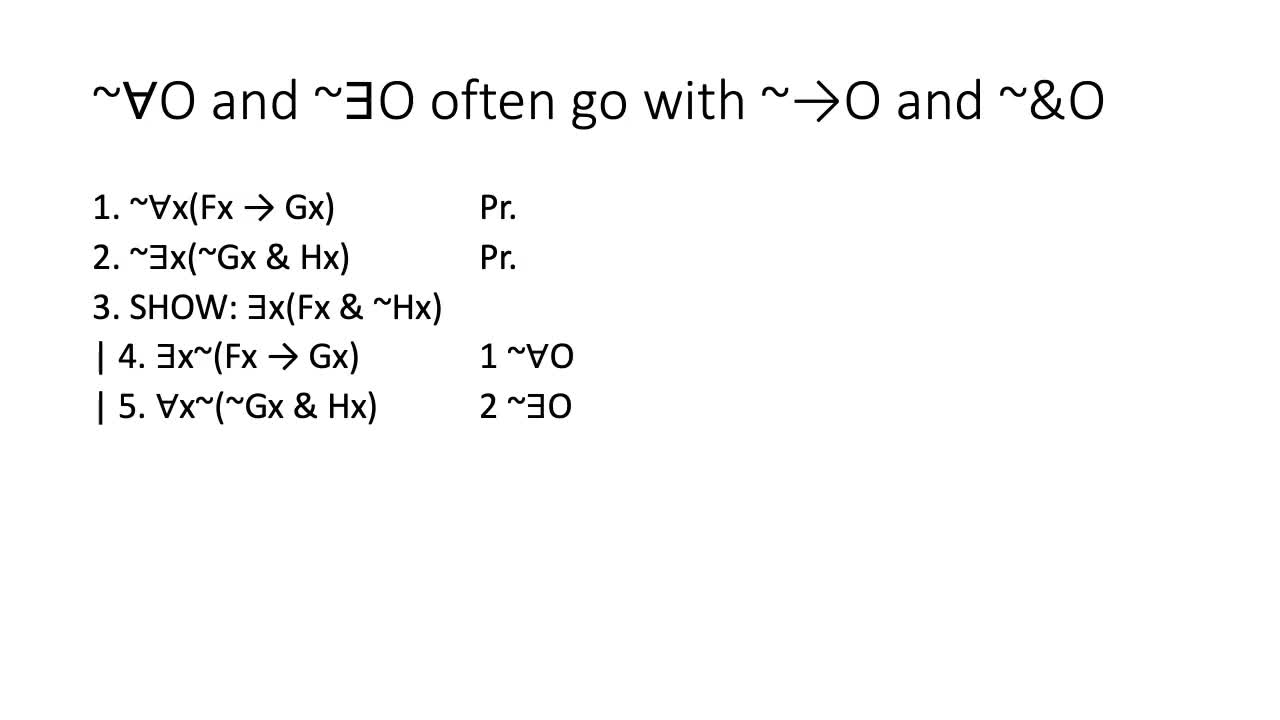9:18

By: mcweiner

A video on how to do proofs with negated quantifiers. Introduces two new rules, ~AO and ~EO, and discusses how to do proofs where you need to SHOW negated quantifiers (you have to do ID).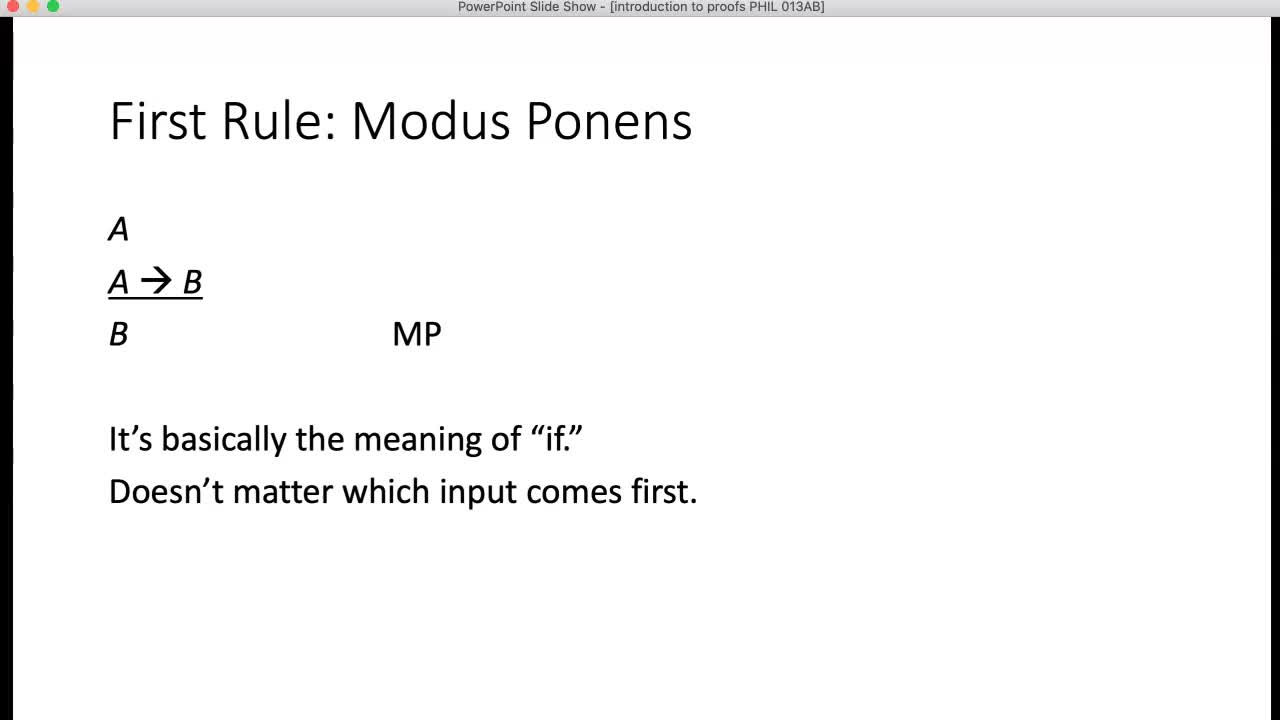8:05

By: mcweiner

A video on what proofs are, which explains the concept of simple derivation, and how to do proofs with one proof rule, Modus Ponens.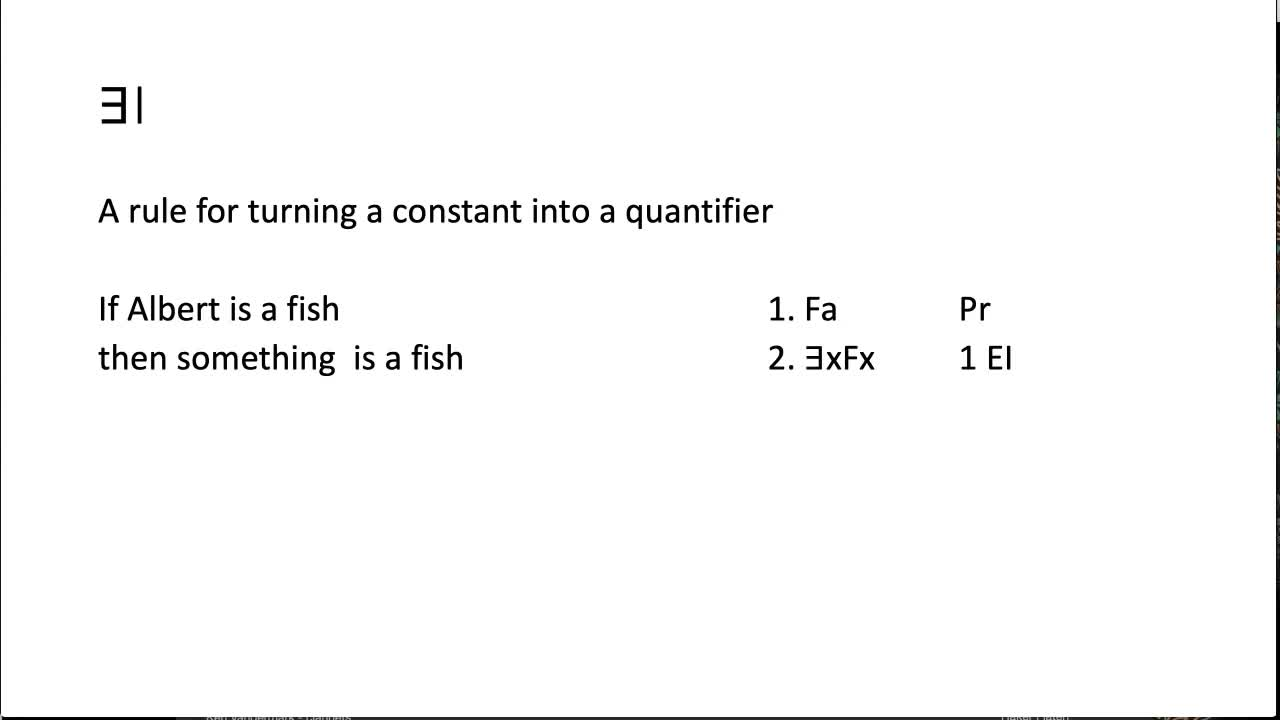7:58

By: mcweiner

A video introducing predicate proofs and the first two predicate proof rules, AO and EI.7:29

By: mcweiner

A video about doing proofs, introducing how to do direct derivations, and the &I and &O rules.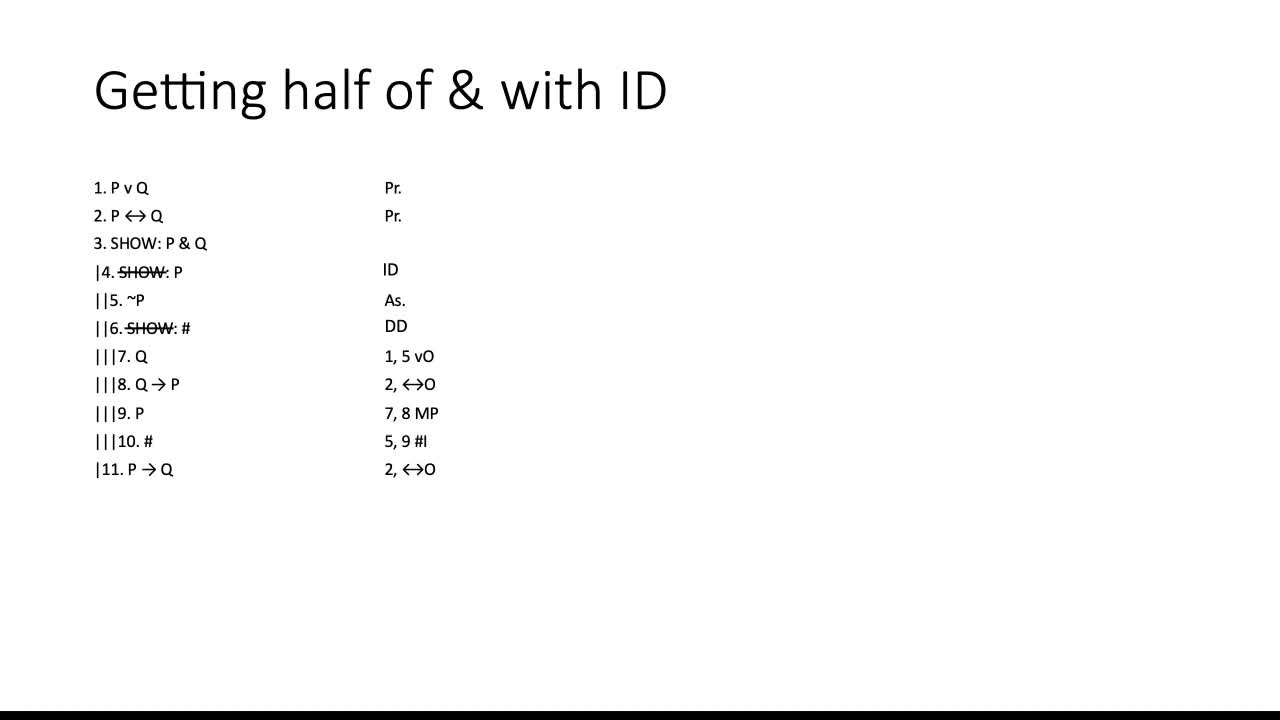7:39

By: mcweiner

A video about how to do proofs where you write down SHOW statements that don't come from any particular derivation rule.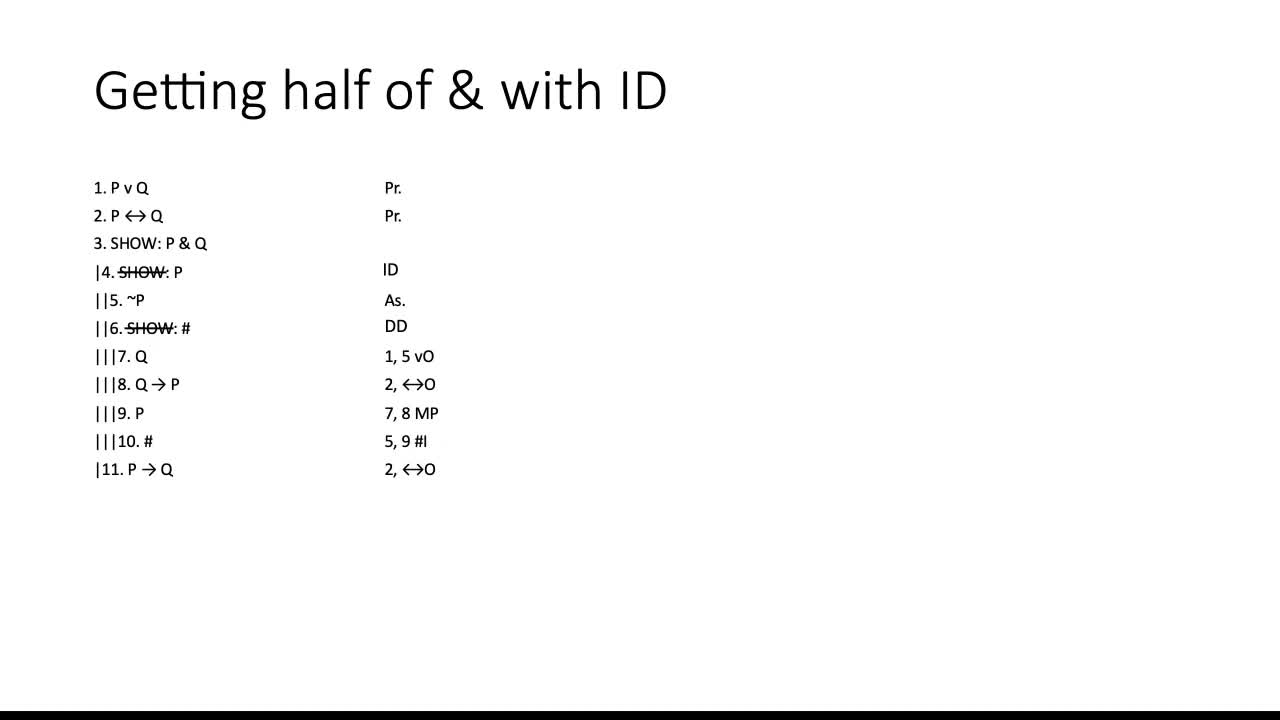7:35

By: mcweiner

A video on how to do proofs where you write down SHOW statements that don't come from specific derivation rules.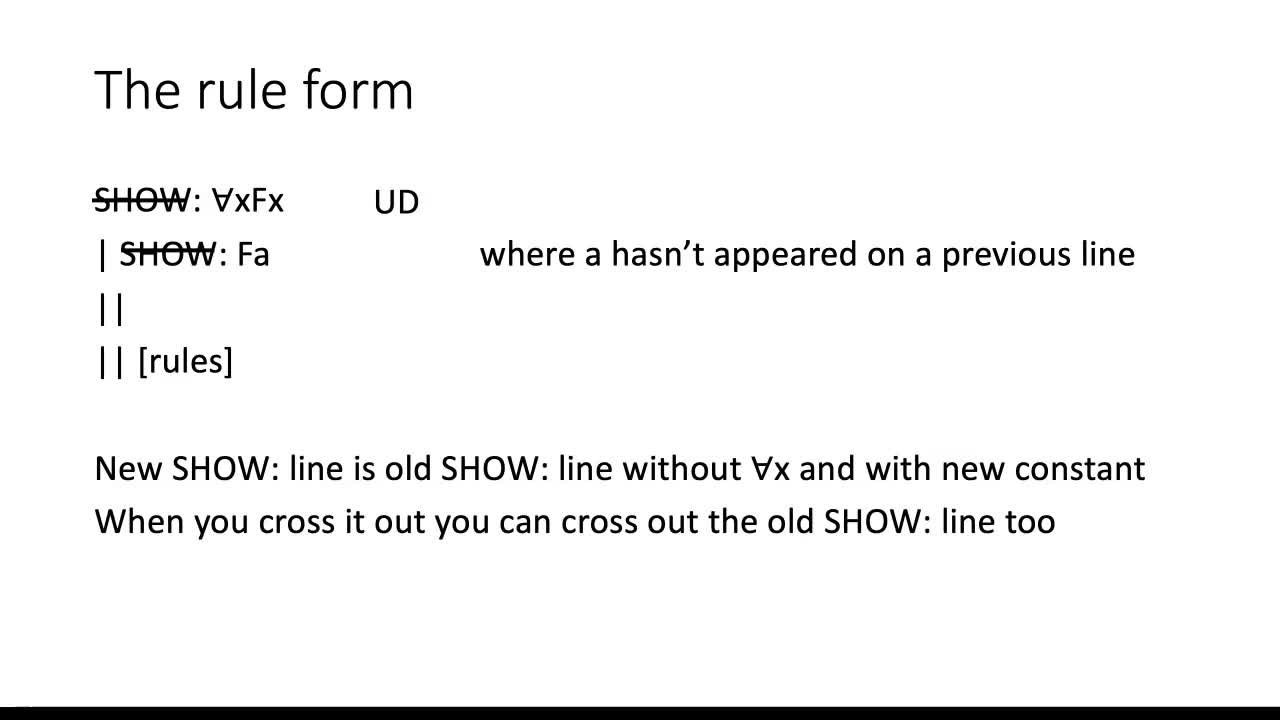5:25

By: mcweiner

A video on the Universal Derivation rule for predicate proofs, a rule for doing proofs where the SHOW line begins with Ax.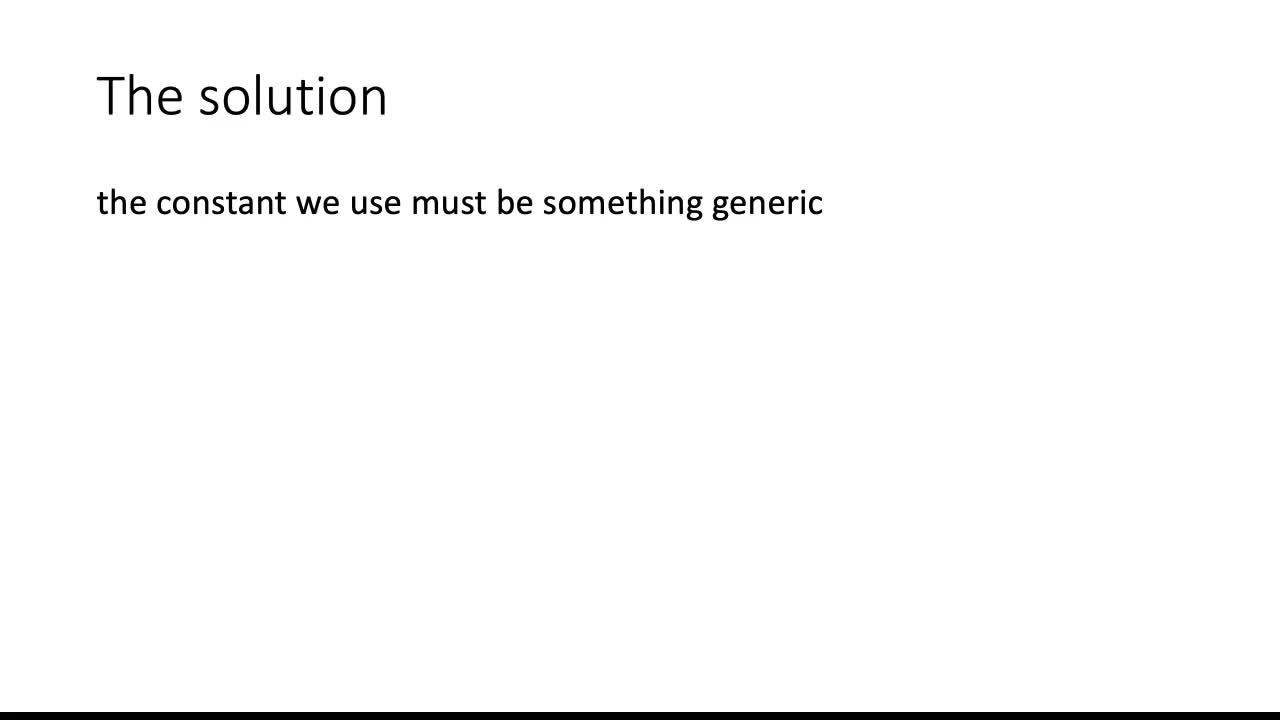7:42

By: mcweiner

A video on how to use the rule EO (existential out) in proofs in predicate logic.